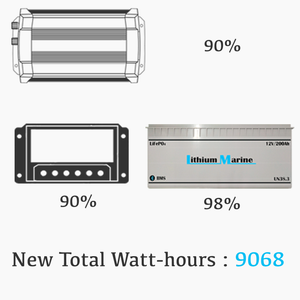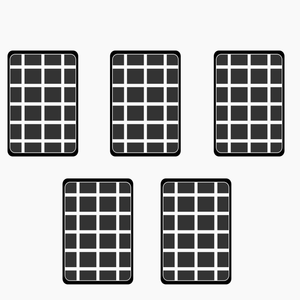A Simple Guide to Off-Grid Solar

A) What are the total Watt-hours needed?

The first step to creating a stand-alone PV system is to calculate your expected power demand in Watt-hours (Wh).

Watt- hours are simply the power draw (Watts) of the appliance(s) x time (Hrs) they will be running at full capacity over a full day, 24Hrs.

Watt-hours = Watts x Hours

The wattage is often displayed as a specification on the relative appliance. If not, it can be found using the voltage and amperage.

Watts = Voltage x AmperageB) Compensate for losses

-Through system components, use their efficiency rating. The AC load is increased due to the inefficiency of the inverter,

= AC demand (Wh) ÷ Efficiency of inverter (%)

= 5984Wh ÷ 90%

= 6649Wh

The DC and AC loads are affected by the Charge controller and battery

= (Adjusted AC load + DC demand) ÷ (Efficiency of battery x  Efficiency of Charge controller)

= (6649Wh + 288Wh) ÷ (90% x 98%)

= 7865Wh2. Solar panels - Supply

A) What is the available sunlight?

The amount of energy from the sun available for solar panels to convert into electricity varies constantly and depends on factors such as cloud cover, angle of the sun to the panels and temperature. “Sun hours” is a value for which these variables are standardised and can be found for your specific location.

According to the Met Office for the month of April England has an average of 6 Sun Hours per day.

B) Minimum Watt-peak (Wp) ?

What is the minimum Wp needed from your solar array to supply 7865Wh under standard conditions given the available sunlight?

Wp needed

= New Total Load ÷ Sun hours

= 7865Wh ÷ 6

= 1310Wp

C) How many Panels?

Solar panels differ in cost, efficiency and construct, the most popular being the polycrystalline silicone wafer type. Cost increases with greater efficiency and higher Watt-peak. For this example we will use 325Wp panels.

Number of panels

= Wp needed ÷ Wp of a single panel

= 1310Wp ÷ 300Wp

= 4.37

5 panels would work well for this system3. Storage - Batteries

A) How many batteries and what arrangement?

Find the Amp-hour (capacity) of storage needed, accounting for DOD (Depth of discharge). Using Our a 200Ah/12.8V battery to 80% DOD,

Ah needed

= New Total Load ÷(DOD x Voltage)

= 7865Wh ÷(80% x 12.8V)

= 768Ah

For this 12VDC/220VAC system 4 batteries of 200Ah connected in parallel to give an 800Ah 12.8V storage bank would be sufficient.

Note: this would provide autonomy over 24Hrs. Operating the appliances for two days without charging would require twice the capacity.4. Inverter

What size inverter?

This is to be matched to the demand (W) of AC appliances, taking into account the efficiency rating of the charge controller.

Inverter size

= Demand of the AC load (W) ÷ efficiency of inverter (%)

= 1012W ÷ 90%

= 1125W

1125W is the smallest inverter that will work in this system. Ensure that the inverters operational voltage is matched to the battery voltage, in this case 12V.5. Charge Controller

What size charge controller?

Changing environmental conditions affect the panels’ voltage and current output. The Maximum power point tracking (MPPT) feature regulates voltage and/or current to ensure the panels are working at their maximum power output. The controller must be matched to the voltage and amperage of the solar array and batteries (note series/parallel arrangement).

Panel specs;

Watt peak = 325

Open circuit voltage (Voc) = 40.8 Volts

Short circuit current (Isc) = 10.41 Amps

5 panels in parallel

= 5 x 10.41A

= 52.05A

Voltage is constant so the minimal requirements for your charge controller are

Amps : 52.05

Volts : 40.8

It is not a problem to oversize the controller with a higher amperage or voltage rating.